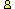CTK Exchange Front Page Movie shortcuts Personal info Awards Reciprocal links Terms of use Privacy Policy Cut The Knot! MSET99 Talk Games & Puzzles Arithmetic/Algebra Geometry Probability Eye Opener Analog Gadgets Inventor's Paradox Did you know?... Proofs Math as Language Things Impossible My Logo Math Poll Other Math sit's Guest book News sit's Recommend this site|Store|CTK Exchange

 Subject: "Combined scaling and rotation" Previous Topic | Next Topic
 ConferencesThe CTK ExchangeCollege mathTopic #508Printer-friendly copyEmail this topic to a friend Reading Topic #508
Ganitopasakguest
May-06-05, 07:14 AM (EST)

"Combined scaling and rotation"

 Hello, I am trying to study combined point transformation. I tried to rotate and then scale a point about origin in a cad package. Here, the sequence does not make any difference. Whether you do scaling first or rotation, the results are correct. Then I set to get the mathematical representations of this. And here is what I have: --- To transform the point P to P’ such that distance from origin is increased by S times and the angle the line joining point with origin is increased by t. Sequence 1: Rotation followed by scaling: Rotation: x’’2 = x1 * cos(t) – y1 * sin(t) y’’2 = x1 * sin(t) + y1 * cos(t) Scaling x2 = Sx * x’’2 y2 = Sy * y’’2 x2 = Sx * (x1 * cos(t) – y1 * sin(t)) y2 = Sy * (x1 * sin(t) + y1 * cos(t)) Sequence 2: Scaling followed by rotation: Scaling X’’2 = Sx * x1 Y’’2 = Sy * y1 Rotation: x2 = x’’2 * cos(t) – y’’2 * sin(t) y2 = x’’2 * sin(t) + y’’2 * cos(t) x2 = Sx * x1 * cos(t) – Sy * y1 * sin(t) y2 = Sx * x1 * sin(t) + Sy * y1 * cos(t) ---- The results are affected by sequence of transformations. Is this correct or have I made a mistake somewhere? If this is correct then what is the source of discrepancy?. If this is correct then can I come to the conclusion that scaling should always be applied before translation? Or non-uniform scaling should be avoided as far as possible? I could not find any convincing solution solution in the couple of books that I have nor on the web. Please help me. =====

sfwc
Member since Jun-19-03
May-06-05, 10:56 AM (EST)1. "RE: Combined scaling and rotation"
In response to message #0

 >The results are affected by sequence of transformations. Is >this correct or have I made a mistake somewhere? If this is >correct then what is the source of discrepancy?. If this is >correct then can I come to the conclusion that scaling >should always be applied before translation? Or non-uniform >scaling should be avoided as far as possible? Under the usual meaning of the term, a scaling would have the same scale factor in all directions. In particular, in your example this would be achieved by setting Sx = Sy. If this is done, you will find that the result you obtain is independent of the order of the transformations. If, however, having Sx not equal to Sy is what you mean by a non-uniform scaling, and this is an essential part of what you are doing, then I can advise you no further without knowing your purpose. So why are you trying to do this?Thankyousfwc<><Ganitopasakguest
May-10-05, 07:00 AM (EST)

2. "RE: Combined scaling and rotation"
In response to message #1

 I am studying Maths required for Solid Modelling (Transformations is a part of it) and preparing detailed notes for my future reference. Thus I need to investigate all possible aspects.alexbCharter Member
1520 posts
May-10-05, 07:02 AM (EST)3. "RE: Combined scaling and rotation"
In response to message #2

 So do that.

 Conferences | Forums | Topics | Previous Topic | Next Topic
 Select another forum or conference Lobby The CTK Exchange (Conference)   |--Early math (Public)   |--Middle school (Public)   |--High school (Public)   |--College math (Public)   |--This and that (Public)   |--Guest book (Public) Educational Press (Conference)   |--No Child Left Behind (Public)   |--Math Wars (Public)   |--Mathematics and general education (Public)You may be curious to have a look at the old CTK Exchange archive.